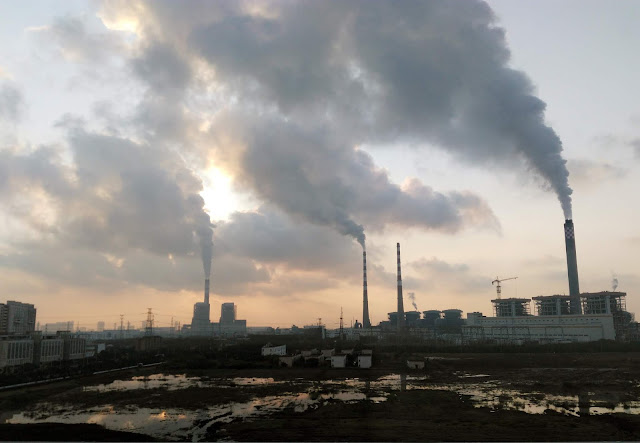# First Law Of Thermodynamics Definition, Equation And Examples

## First Law Of Thermodynamics DefinitionWorking Of A Thermal Power Station Is Based On 1st Law Of Thermodynamics Credit: Wikimedia Commons

The First Law Of Thermodynamics is one of the Physical Laws Of Thermodynamics (other are Zeroth Law, 2nd Law, and 3rd Law) that states that heat is a form of energy and the total energy of a system and it’s surrounding remained conserved or constant. Or in more simple terms, for an isolated system; energy can neither be created nor be destroyed. It can only be converted from one form of energy to another. That’s why the 1st law of thermodynamics is also known as the Law Of Conservation Of Energy. This law was discovered by German mathematician and physicist Rudolf Clausius.

According to this law, heat energy can only be transformed from one system to another. That’s why it is being said that Thermodynamics and Heat Transfer go hand in hand. On the other hand, with the discovery of the First Law Of Thermodynamics, it was confirmed that it is impossible to build the Perpetual Motion Machines Of First Kind. Or in more simple terms, no machine can work without using a power source. And if it does, then it will directly violate the first law of thermodynamics.

## First Law Of Thermodynamics Equation

According to the First Law Of Thermodynamics Equation, there are three parameters involved
• ΔU  —  Change in Internal Energy of the system
• W    —  Work is done ON or BY the system
• Q     —  Heat supplied to the system

The equation of this law can be written in two way:

### When Work Is Done By The System

ΔU = Q – W
Here ΔU denotes the change in the internal energy of a closed system. Q denotes the heat supplied to the closed system. And W denotes the amount of useful work done by the system. This version of the first law of thermodynamics equation was given by the old guard of classical thermodynamics i.e Rudolf Clausius. This type of equation is generally used for the applications of Heat Engines.

### When Work Is Done On The System

ΔU = Q + W

Nowadays IUPAC uses the sign convention given by the new guard i.e Max Planck. As Max Planck stated that according to the above equation, the net energy transferred to the system is positive, and on the other hand, net energy transferred from the system is negative. W denotes the amount of useful work done on the system.

## First Law Of Thermodynamics Example

Well, all I can say that every man-made machine we see around us is directly or indirectly an example of the First Law. Even a heaven-made machine that we see daily i.e The Sun is an example of the first law. Whatever we do in our day-to-day life such as cooking, riding a bicycle, working of an air conditioner, fan, refrigerator, or simply speaking eating food is an application of this law.

Basically, the first law’s examples can be classified into two categories:-
• when work is done on the system
• when work is done by the system

Working of a Refrigerator is an example of when work is done on the system. And, on the other hand, working of heat engines like a Thermal Power Plant or Internal Combustion Engines is an example of when work is done by the system.

That’s it for this post. If you like this article, share it if you like it, like it if you share it. You can also find us on Mix, Twitter, Pinterest, and Facebook.